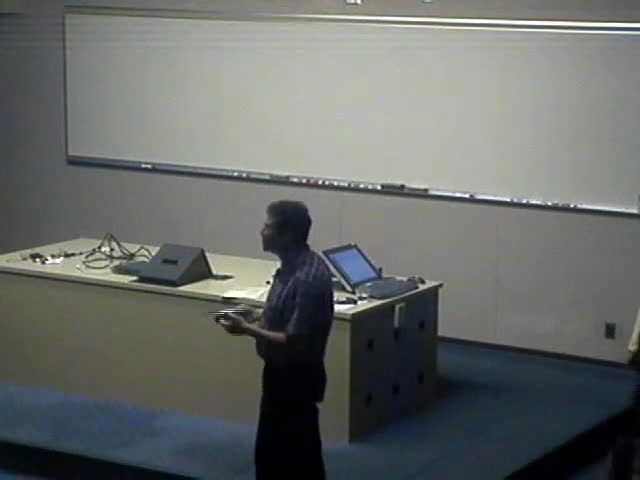### Videos

#### Mechanisms and Robot Kinematics: Numerical Algebraic Geometry

##### Presenter
September 16, 2006##### Abstract
This talk will discuss how numerical polynomial continuation can be used to solve the problems formulated in the first lecture. In doing so, we will describe the basic constructs and algorithms of Numerical Algebraic Geometry. Foremost among these is the notion of a witness set, a numerical approximation to a linear section of an algebraic set. We will describe how witness sets are computed, how they are used in finding numerical irreducible decompositions, and how the witness set for the intersection of two algebraic sets, say A and B, can be found from the witness sets for A and B, via a diagonal homotopy. Some recent avenues of research, such as how to find the real solutions inside a complex curve, will be mentioned briefly. Suggested reading: A.J. Sommese and C.W. Wampler, The numerical solution of systems of polynomials arising in engineering and science, World Scientific, 2005.